# The Most Amount of Rain over a 10 Day Period on Record

February 14, 2019
By

Want to share your content on R-bloggers? click here if you have a blog, or here if you don't.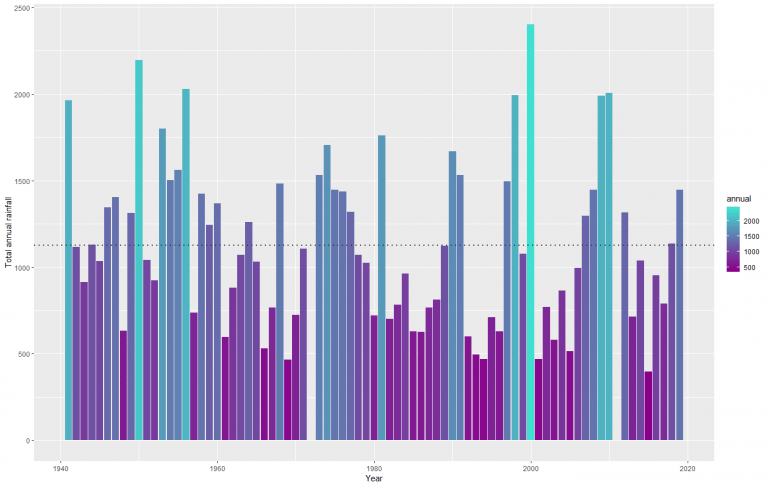Townsville, Qld, has been inundated with torrential rain and has broken the record of the largest rainfall over a 10 day period. It has been devastating for the farmers and residents of Townsville. I looked at Townsville’s weather data to understand how significant this event was and if there have been comparable events in the past.

## Data from ‘bomrang’

Where this may interest the R community is in obtaining the data. The package ‘bomrang’ is an API allowing R users to fetch weather data directly from the Australian Bureau of Meteorology (BOM) and have it returned in a tidy data frame.

Historical weather observations including rainfall, min/max temperatures and sun exposure are obtained via `get_historical()`. Either the ID or the lat-long coordinates of the weather station are needed to extract the data. The ID information can be found on the BOM website by navigating to the observations page.

Using the station ID the rainfall data is extracted with the following.

```suppressPackageStartupMessages(library(tidyverse))
suppressPackageStartupMessages(library(bomrang))
suppressPackageStartupMessages(library(gridExtra))
suppressPackageStartupMessages(library(magrittr))
suppressPackageStartupMessages(library(MCMCpack))
mycols <- c("darkmagenta", "turquoise")

# import data - simple as
townsville <- get_historical("032040")```

And cleaning up the dates.

```# fix date functions
fix.date <- function(y,m,d){
s <- paste(c(y, m, d), collapse = "-")
d <- as.Date(s, "%Y-%m-%d")
return(d)
}
for(k in 1:nrow(townsville)){
townsville\$date[k] <- fix.date(townsville\$Year[k], townsville\$Month[k], townsville\$Day[k])
}
townsville\$date <- as.Date(townsville\$date, origin = "1970-01-01")

# trim - for replication of when this was first run
townsville %<>% dplyr::filter(date < as.Date("2019-02-13"))

# take a look

```##   Product_code Station_number Year Month Day Rainfall Period Quality
## 1   IDCJAC0009          32040 1941     1   1      0.0     NA       Y
## 2   IDCJAC0009          32040 1941     1   2      6.6      1       Y
## 3   IDCJAC0009          32040 1941     1   3     16.5      1       Y
## 4   IDCJAC0009          32040 1941     1   4    205.5      1       Y
## 5   IDCJAC0009          32040 1941     1   5    175.0      1       Y
## 6   IDCJAC0009          32040 1941     1   6     72.9      1       Y
##         date
## 1 1941-01-01
## 2 1941-01-02
## 3 1941-01-03
## 4 1941-01-04
## 5 1941-01-05
## 6 1941-01-06```

## Total rainfall over a 10 day period

Applying a 10 day rolling window over the entire historical record it’s easy to see the significance of this rainfall event. The 8th February recorded 1259.8mm of rain in the 10 days prior. It dwarfs the previous record of 925.5mm set in 1953. It also highlights other significant events in the past, in particular 1968, 1998 and 2009 but these don’t come close to the 2019 event.

```# get 10 day total
townsville\$rolling10 <- 0
for(k in 10:nrow(townsville)){
townsville\$rolling10[k] <- sum(townsville\$Rainfall[(k-9):k])
}

# plot
ggplot(
townsville %>% dplyr::filter(date > as.Date("1940-01-01")),
aes(x = date, y = rolling10, col = rolling10)) + geom_line() +
labs(y = "Total rainfall in the last 10 days")```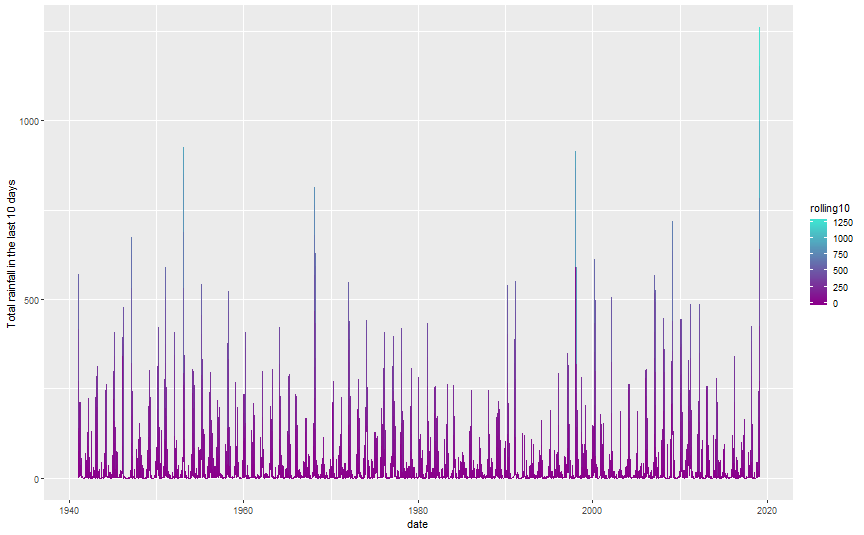It really was a phenomenal amount of rain. This was not the largest rainfall in a day however, that record occurred in 1998 with a massive 548.8mm of rain. In fact the 2019 floods don’t feature in the top 10 wettest days, but the consistency over 10 days made it the wettest on record.

`townsville %>% arrange(desc(Rainfall)) %>% dplyr::select(date, Rainfall) %>% head(10)`

```##          date Rainfall
## 1  1998-01-11    548.8
## 2  1946-03-03    366.5
## 3  1953-01-16    346.7
## 4  1977-02-01    317.6
## 5  1997-03-24    302.8
## 6  1978-01-31    273.4
## 7  2000-04-04    271.6
## 8  1946-02-10    251.7
## 9  2009-02-03    236.8
## 10 1944-03-29    233.4```

## Annual rainfall pattern

Townsville received over a years worth of rain in 10 days. The graph below shows the annual rainfall measurements and average annual rainfall (dotted line) given the historical records. Even with only 5-6 weeks of the year, 2019 is already one of the wettest years on record.

```# calculate the total annual rainfall and rainfall to date
annual.rainfall <- townsville %>%
dplyr::filter(date > as.Date("1940-01-01")) %>%
mutate(
rainfall_to_date = as.numeric(as.POSIXlt(date)\$yday < 40)*Rainfall,
rainfall_after_wet = as.numeric(as.POSIXlt(date)\$yday < 90)*Rainfall
) %>%
group_by(Year) %>%
summarise(
annual = sum(Rainfall),
Feb12th = sum(rainfall_to_date),
april = sum(rainfall_after_wet),
remaining = sum(Rainfall) - sum(rainfall_to_date)
)

# bar plot
ggplot(annual.rainfall, aes(x = Year, y = annual, fill = annual)) +
geom_bar(stat = "identity") +
geom_hline(yintercept = mean(annual.rainfall\$annual, na.rm = TRUE), lty = 3, col = "grey20", lwd = 1) +
labs(y = "Total annual rainfall")```On close inspection the data suggests that the first 40 years of records are less variable than from 1980 on-wards. There appears to be drier years and wetter years in the latter half.

## Record annual rainfall in 2019?

The current record was set in 2000 at 2400mm where in this year Townsville had a few heavy rainfall events in the months up until April and some lesser events near the end of the year. Comparing 2019 to these years, there is definitely potential for 2019 to be the wettest on record.

```ggplot(townsville %>% dplyr::filter(Year %in% c(1950, 1956, 2000, 2019)), aes(x = as.POSIXlt(date)\$yday, y = Rainfall)) +
geom_line() +
facet_grid(Year ~ .) + labs(x = "Day of the year") +
labs(title = "Wettest 3 years on record vs 2019")```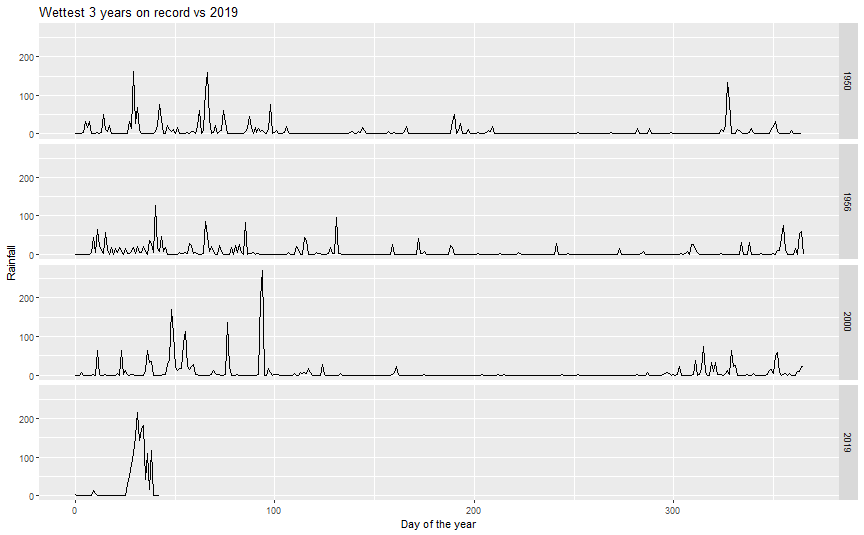Below clearly shows which years have had significant rainfall in the first part of the year. The years which have received greater than 700mm (dotted line) are quite distinct from the bulk of the data. Since the wet season ends in April the other heavy years (like 2000) haven’t had their major events yet. This is shown in the April plot at the bottom which has a much stronger relationship (obviously). The years which experienced heavy rainfall at this time of year, in general didn’t get too much afterwards.

```grid.arrange(
ggplot(annual.rainfall, aes(x = Feb12th, y = annual, col = annual)) +
geom_point(size = c(rep(2, nrow(annual.rainfall)-1), 4)) +
labs(y = "Annual rainfall", x = "Total rainfall as at 12th Feb") +
geom_vline(xintercept = 700, lty = 3, col = "grey20", lwd = 1),
ggplot(annual.rainfall, aes(x = april, y = annual, col = annual)) +
geom_point(size = c(rep(2, nrow(annual.rainfall)-1), 4)) +
labs(y = "Annual rainfall", x = "Total rainfall as at 1st April") +
geom_vline(xintercept = 700, lty = 3, col = "grey20", lwd = 1)
)```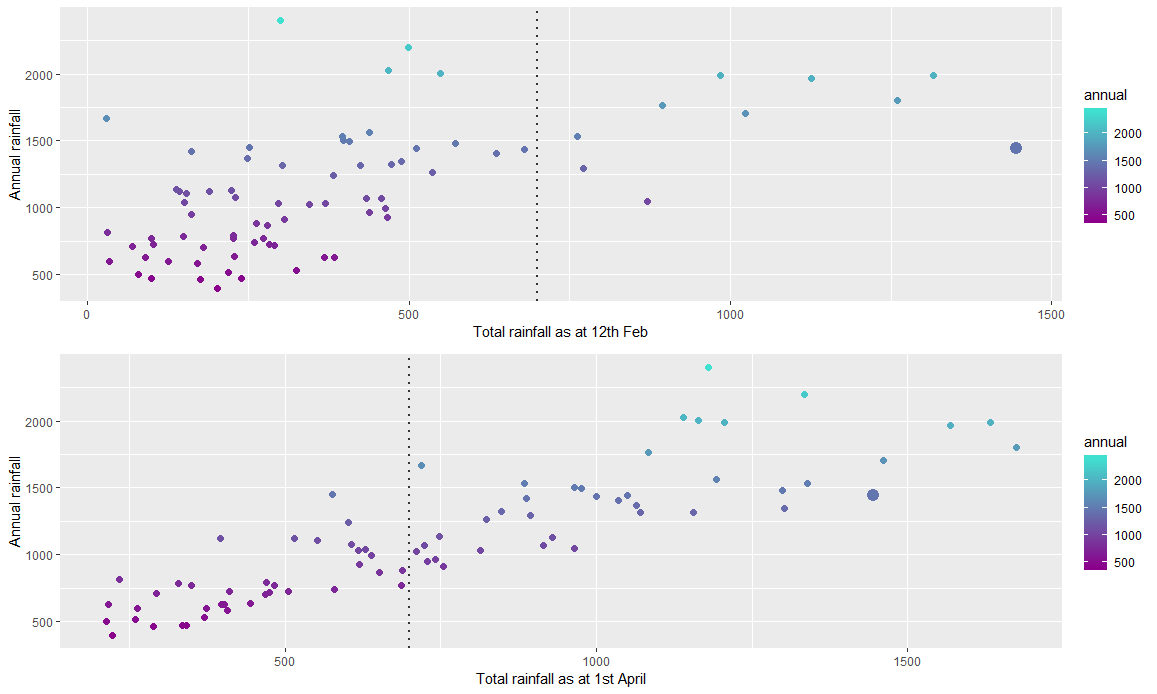For what it’s worth I’ll offer a prediction for the expected annual rainfall and probability of being the wettest year on record (which, to be honest is a fools errand – tropical weather systems are pretty complex stuff)

```# bayesian model
blm <- MCMCregress(annual ~ Feb12th, data = annual.rainfall %>% dplyr::filter(Feb12th > 0, Year != 2019),
sigma.mu = 235, sigma.var = 35^2) # priors from exploration - details skipped here

# prediction
x <- matrix(c(1, 1444))
pred.annual.rainfall <- data.frame(
annual = blm[,-3] %*% x + rnorm(10000, 0, sqrt(blm[,"sigma2"])), # posterior predictive distribution
exp.val = blm[,-3] %*% x) # mean distribution
# c(min(pred.annual.rainfall\$annual), table(pred.annual.rainfall\$annual < 1444)/10000)

n <- 1000
xstart <- rep(0, n)
xend <- rep(1700, n)
ystart <- blm[1:n,1] + blm[1:n,2]*xstart
yend <- blm[1:n,1] + blm[1:n,2]*xend
ystartp <- blm[1:n,1] + blm[1:n,2]*xstart + rnorm(n, 0, sqrt(blm[,3]))
yendp <- blm[1:n,1] + blm[1:n,2]*xend + rnorm(n, 0, sqrt(blm[,3]))
df.seg <- data.frame(xstart, xend, ystart, yend, ystartp, yendp)

exp.val <- quantile(pred.annual.rainfall\$exp.val, c(0.025, 0.5, 0.975))
post.pred <- quantile(pred.annual.rainfall\$annual, c(0.025, 0.5, 0.975))
prob.record.rainfall <- sum(as.numeric(pred.annual.rainfall\$annual > 2400))/10000

# drawing each line of the posterior draws takes time but its a nice aesthetic
df.pred <- data.frame(x = c(1444, 1444), y = range(exp.val))
a <- 6
df.post <- data.frame(xs = rep(1444, 3) + c(0, -a, -a), xe = rep(1444, 3) + c(0, a, a),
ys = c(post.pred, post.pred, post.pred), ye = c(post.pred, post.pred, post.pred))
ggplot(annual.rainfall %>% dplyr::filter(Year != 2019, Feb12th > 0), aes(x = Feb12th, y = annual)) +
geom_segment(mapping = aes(x = xstart, xend = xend, y = ystartp, yend = yendp), data = df.seg, col = "grey20", alpha = I(0.05)) +
geom_segment(mapping = aes(x = xstart, xend = xend, y = ystart, yend = yend), data = df.seg, col = "darkmagenta", alpha = I(0.025)) +
geom_point() +
labs(y = "Annual rainfall", x = "Total rainfall as at 12th Feb") +
geom_line(mapping = aes(x = x, y = y), data = df.pred, size = 2) +
geom_segment(mapping = aes(x = xs, xend = xe, y = ys, yend = ye), data = df.post) +
geom_hline(yintercept = 2400, col = "red", lty = 2)```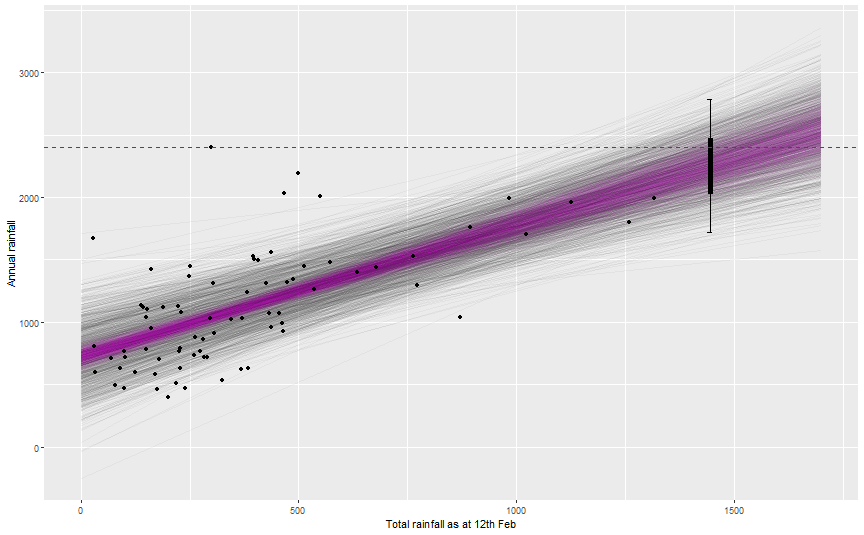Based on this (crude) model, the expected annual rainfall credible interval is (2027, 2472) mm. Using the posterior predictive distribution for 2019, the probability 2019 will experience record rainfall is 0.29.

But let’s all hope there’s not too much more rain. There has been enough.

The post The Most Amount of Rain over a 10 Day Period on Record appeared first on Daniel Oehm | Gradient Descending.

R-bloggers.com offers daily e-mail updates about R news and tutorials about learning R and many other topics. Click here if you're looking to post or find an R/data-science job.
Want to share your content on R-bloggers? click here if you have a blog, or here if you don't.